New Students Offer - Use Code HELLO

# Profit and Loss 20 Repeated Questions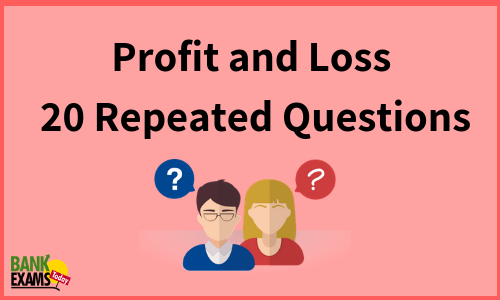Profit and loss is an important chapter for competitive examinations. From past few years I am analyzing question papers of banking examinations. Today I am sharing 20 questions that are repeated in the exams.

#### Ques 1.

I sold two hens for Rs. 105 each . On one I lost 25% . On the other I gained 50% . What is the gain or loss on my total outlay ?
Ans 1.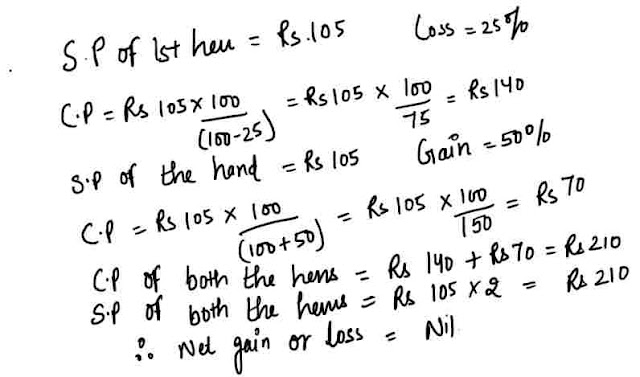#### Ques 2.

Sudhira sold a watch at a profit of 20%. Had she bought it at 10%less and sold it at Rs 30 less, she would still have gained 20% .Find the cost price of the watch.
Ans 2. Let the C.P.  of the watch be Rs x.
Gain % in both the cases is the same .Hence, C.P.  of the watch = Rs.250.

#### Ques 3.

I bought eggs at the rate of 5 for Rs 7 and sold them at the rate of 7 for Rs. 10 and made Rs.2450 in the bargain. How many eggs did I deal in ?
Ans 3. Suppose I bought 35 eggs.
C.P. of 35 eggs = Rs. 49, S.P. of 35 eggs = Rs 50
Profit on 35 eggs = Re 1

∴ required number of eggs = 2450/1 x 35 = 85750.

#### Ques 4.

A shop's prices are 25% above cost price, but it allows its customers 12% commission on the amounts of their bills. What is the profit per cent made ?
Ans 4.#### Ques 5.

Suruchi sold two pens at Rs.20 each . Find her common loss or gain % if on pen she gains 10% and on the other suffers a loss of 10%.
Ans 5.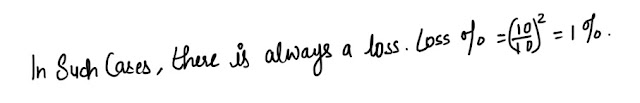#### Ques 6.

A dishonest retailer professes to lose 4% on tea but uses a false weight equal to 9000 g instead of 1Kg. Find his loss or gain %.
Ans 6.#### Ques 7.

A man buys oranges at a certain price per dozen and sells them at eight times that price per hundred . What is his gain or loss % ?
Ans 7. Let C.P. of 12 oranges = Re 1
S.P. of 100 oranges = Rs 8#### Ques 8.

I sold a book at a profit of 12%. Had I sold it for 18 more, 18% would have been gained . find the cost price.
Ans 8.#### Ques 9.

The cost price of 24 articles is equal to the selling price of 18 articles . Find the gain per cent .
Ans 9.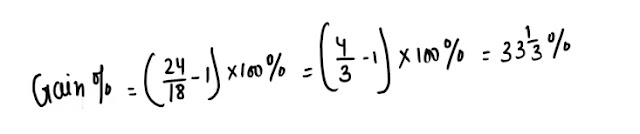#### Ques 10.

How much per cent above the cost price must a trader mark his goods so as to earn a profit of 36% after allowing a discount of 15 % on the marked price ?
Ans 10. Let  the required percentage be  x %.Hence, the trader must mark his goods 60 % above  the cost price .

#### Ques 11.

A shopkeeper sells an item at a profit of 20%. Find the ratio of CP and SP.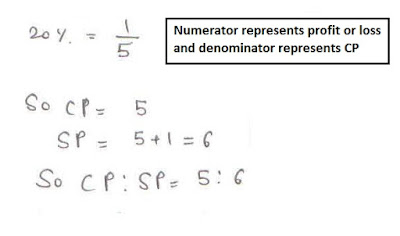#### Ques 12.

A shopkeeper sells an item a profit of. If the cost price of the item was Rs.696, find the selling price of the item.
Ans 12.#### Ques 13.

The selling price of a horse is Rs.3618. It was sold at a loss ofFind the cost price of the horse.
Ans 13.#### Ques 14.

The sum of selling price and cost price of a bicycle is Rs.9300. If the bicycle is sold at a loss of, find the selling price of the bicycle.
Ans 14.#### Ques 15.

A shopkeeper sold an item at a profit ofon S.P. Find the actual profit percentage.
Ans 15.#### Ques 16.

A shopkeeper sold an item at a loss ofon SP. If the sum of CP and SP of the article is Rs.1768, find the SP of the article.
Ans 16.#### Ques 17.

An article was sold at a price of Rs.500 at a profit of 25%. Find the SP of the article, if the profit percentage is doubled.
Ans 17.#### Ques 18.

A shopkeeper sold an article at Rs.480 at a loss of. Find the SP of the article with 25% profit.
Ans 18.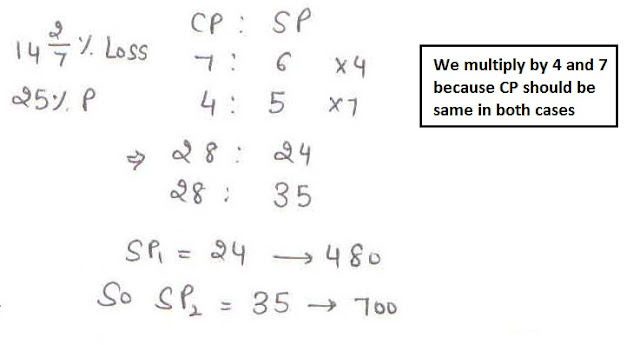#### Ques 19.

A pen was sold atloss. If the same article was sold atprofit then it would have fetched Rs.100 more. Find the cost price of the article.
Ans 19.#### Ques 20.

The difference between the selling price of an article when it is sold at a profit of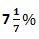and a loss ofis Rs. 2700. Find the cost price of the article.
Ans 20.